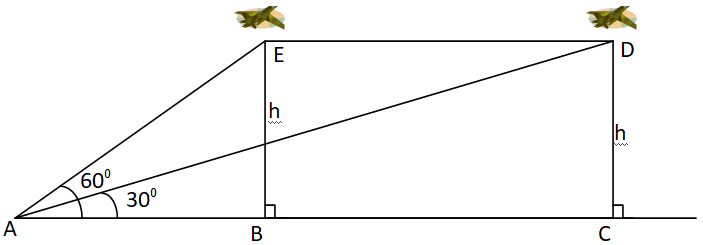Courses
Courses for Kids
Free study material
Free LIVE classes
MoreLIVE
Join Vedantu’s FREE Mastercalss

# The angle of elevation of an aeroplane from a point on the ground is ${60^0}$. After a flight of 30 seconds the angle of elevation becomes ${30^0}$ . If the aeroplane is flying at a constant height of $3000\sqrt 3 {\text{ }}m$. Find the speed of the aeroplane.Verified
361.5k+ views
Hint- Use the definition of angle of elevation and simple formula of tangent, and then proceed further by using the basic formula of speed as distance upon time.We will use the above diagram to solve the problem.
Let $E{\text{ and }}D$ be the two positions of the plane and $A$ be the point of observation.
Let $ABC$ be the horizontal line through $A$ .
It is given that angles of elevation of the plane in two positions $E{\text{ and }}D$ from the point $A$ are ${\text{6}}{{\text{0}}^0}{\text{ and 3}}{{\text{0}}^0}$ respectively.
$\angle EAB = {60^0},\angle DAB = {30^0}$
It is also given that $EB = DC = 3000\sqrt 3 {\text{ }}m$
As we know that in any right angled triangle $\tan \theta = \dfrac{{{\text{height }}}}{{{\text{base}}}}$
$\therefore {\text{ In }}\Delta ABE,\tan \left( {\angle EAB} \right) = \dfrac{{BE}}{{AB}} \\ \Rightarrow \tan \left( {{{60}^0}} \right) = \dfrac{h}{{AB}} \\ \Rightarrow \dfrac{{\sqrt 3 }}{1} = \dfrac{{3000\sqrt 3 }}{{AB}}{\text{ }}\left[ {\because \tan \left( {{{60}^0}} \right) = \sqrt 3 } \right] \\ \Rightarrow AB = 3000m \\$
Similarly,
$\therefore {\text{ In }}\Delta ACD,\tan \left( {\angle DAC} \right) = \dfrac{{DC}}{{AC}} \\ \Rightarrow \tan \left( {{{30}^0}} \right) = \dfrac{h}{{AC}} \\ \Rightarrow \dfrac{1}{{\sqrt 3 }} = \dfrac{{3000\sqrt 3 }}{{AC}}{\text{ }}\left[ {\because \tan \left( {{{30}^0}} \right) = \dfrac{1}{{\sqrt 3 }}} \right] \\ \Rightarrow AC = \left( {3000\sqrt 3 \times \sqrt 3 } \right)m \\ \Rightarrow AC = 9000m \\$
Now the distance travelled by the aeroplane according to the figure is $BC$ .
$\Rightarrow BC = AC - AB \\ \Rightarrow BC = 9000m - 3000m \\ \Rightarrow BC = 6000m \\ \Rightarrow BC = 6km{\text{ }}\left[ {\because 1000m = 1km} \right] \\$
Therefore, the plane covers $6km$ in $30$ seconds.
So the speed of the plane is
${\text{speed = }}\dfrac{{{\text{distance}}}}{{{\text{time}}}} \\ \Rightarrow {\text{speed}} = \dfrac{{6000m}}{{30{\text{second}}}} \\ \Rightarrow {\text{speed}} = 200\dfrac{m}{{\sec }} \\$
Hence, the speed of the aeroplane is $200\dfrac{m}{{\sec }}$ .

Note- In order to solve such questions of height and distances, figures are a must to start the solution and also for better understanding. Trigonometric values at some particular angles such as ${30^0},{60^0},{90^0}....$ must be remembered. Also take special care of units at the time of finding speed of some moving object.
Last updated date: 26th Sep 2023
Total views: 361.5k
Views today: 9.61k